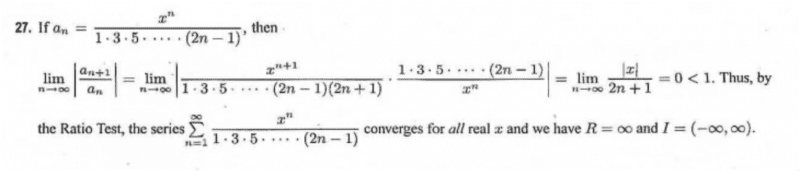# Applying the ratio test

Below is a screen shot of a solution to a problem. The part I don't fathom is after the ratio test is applied to the denominator. How can, noting that an+1, (2n-1) become (2n-1)(2n+1) and not just (2(n+1)-1)=2n+1?

Thank you in advance## Answers and Replies

Mark44
Mentor
Below is a screen shot of a solution to a problem. The part I don't fathom is after the ratio test is applied to the denominator. How can, noting that an+1, (2n-1) become (2n-1)(2n+1) and not just (2(n+1)-1)=2n+1?

Thank you in advance
View attachment 83898
In an, the denominator is the product ##1 \cdot 3 \cdot 5 \cdot \dots \cdot (2n - 1)##. What will be the next factor in the denominator for an + 1?

BTW, when you post a question here, please don't delete the three parts of the homework template.

•shanepitts
In an, the denominator is the product ##1 \cdot 3 \cdot 5 \cdot \dots \cdot (2n - 1)##. What will be the next factor in the denominator for an + 1?

BTW, when you post a question here, please don't delete the three parts of the homework template.

Thanks for the quick reply

Asked in that manner, the next part is 2n+1. But why not just replace the n with (n+1)? And why would the answer be completely different if this approach to problem is taken?

Mark44
Mentor
Thanks for the quick reply

Asked in that manner, the next part is 2n+1. But why not just replace the n with (n+1)? And why would the answer be completely different if this approach to problem is taken?
It wouldn't. If you replace n by n + 1 in the expression 2n - 1, what do you get?

•shanepitts
It wouldn't. If you replace n by n + 1 in the expression 2n - 1, what do you get?

You get 2n+1 instead of the (2n-1)(2n+1), no?

Mark44
Mentor
You get 2n+1 instead of the (2n-1)(2n+1), no?
You get (2n + 1). The (2n - 1) factor is the one from an.

•shanepitts
You get (2n + 1). The (2n - 1) factor is the one from an.

Thanks,
I'm sorry but I still don't fathom.

Let's say

αn=2n-1 then αn+1 should equal 2(n+1)-1=2n+1

Why the extra 2n-1 in the αn+1?

Mark44
Mentor
Thanks,
I'm sorry but I still don't fathom.

Let's say

αn=2n-1 then αn+1 should equal 2(n+1)-1=2n+1
This is not an, at least as it's defined in post 1.

$$a_n = \frac{x^n}{1 \cdot 3 \cdot 5 \cdot \dots \cdot (2n - 1)}$$

Now, what is an + 1?
You need to ask yourself how many factors are in the denominator of an? How many are in the denominator of an + 1?
shanepitts said:
Why the extra 2n-1 in the αn+1?

•shanepitts